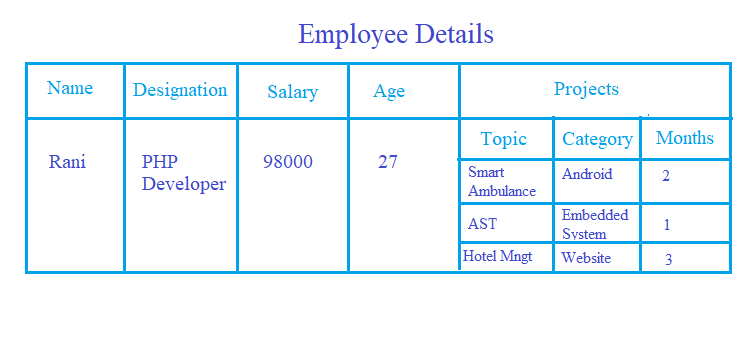# How to convert JSON to XML using Python

In this article, we will study how to convert JSON to XML using Python. Let us quickly understand what is JSON and XML.

JSON stands for JavaScript Object Notation. JSON stores and exchange the data. Hence, JSON is a plain text. In Python, JSON is a built-in package. A JSON file is a file that stores data in JavaScript Object Notation (JSON) format. JSON is easy to understand.

XML stands for eXtensible Markup Language. Similar to JSON, even XML stores and exchange the data.XML is a markup language. It defines set of rules which are used to encode the documents in such a way that it is readable to both machine as well as human. XML is self-descriptive.

Hence, JSON  is a way of representing objects and XML is a markup language.

Let us now create a JSON file. Following are the steps to create JSON File:

STEP 1: Gather Data

We have to gather the required data first to create JSON file. Let us create JSON file for an employee. Hence, we will gather data of an employee.Step2: Write JSON Code

We will write a JSON Code for the above data. Look at the following code:

```{
"Name" : "Rani",
"Designation" : "PHP Developer",
"Salary" : 98000,
"Age":27,
"Projects" : [
{"Topic":"Smart Ambulance","Category":"Android Application","Months":2},
{"Topic":"AST","Category":"Embedded System","Months":1},
{"Topic":"Plant Nursery","Category":"Website","Months":3}
]
}```

Step3: Create JSON file

Open a blank file. Copy the above code and the save it with “.json” extension. Let us save this file as “employee_json_file.json”. Hence, we have created JSON file.

## Conversion of JSON to XML in Python

Let us now convert JSON to XML using Python. We will understand the conversion step by step.

(i) Import JSON

Python has a built-in package “json”. Whenever we want to work with json data in python we have to import this package. Look at the following line:

`import json as j`

We will open our JSON file which we have created and load its data in a variable using “json.load()”. Look at the following code:

```with open("employee_json_file.json") as json_format_file:

(iii) Import xml.etree.cElementTree

We will now import the xml library xml.etree.cElementTree. This library is used to create XML file. Look at the following line:

`import xml.etree.cElementTree as e`

(iv) Create root

We will now create root of XML file. An XML file must contain a root. Each XML file has exactly one root element. An XML file without root element is considered invalid. Look at the following code:

`r = e.Element("Employee")`

Here “Employee” is the name of root.

(v) Create SubElement

We will create SubElements of root for our XML Documents. Look at the following code:

```e.SubElement(r,"Name").text = d["Name"]

e.SubElement(r,"Designation").text = d["Designation"]

e.SubElement(r,"Salary").text = str(d["Salary"])

e.SubElement(r,"Age").text = str(d["Age"])

project = e.SubElement(r,"Projects")

for z in d["Projects"]:
e.SubElement(project,"Topic").text = z["Topic"]
e.SubElement(project,"Category").text = z["Category"]
e.SubElement(project,"Months").text = str(z["Months"])```

Here, “d” is the variable in which we have loaded our JSON data. The “text” is used to assign value.

SubElement takes two parameter:

• root- It is name of the variable where root element is stored.
• subelement_name: It is the name of subelement.

Also, we have converted “Salary”,”Age” and “Months” from integer to string because in XML we cannot store integer or float objects.

(vi) Build XML Tree

Now we will create XML Tree. Look at the following code:

`a = e.ElementTree(r)`

(vii) Create XML File

Finally we will create XML file. We have to pass the path of file where this XML file will be saved. Look at the following code:

`a.write("json_to_xml.xml")`

In the location which you have given, search for the XML file. The JSON data would have been converted into XML.

Entire code is as follow:

```import json as j

with open("json_file.json") as json_format_file:

import xml.etree.cElementTree as e

r = e.Element("Employee")

e.SubElement(r,"Name").text = d["Name"]

e.SubElement(r,"Designation").text = d["Designation"]

e.SubElement(r,"Salary").text = str(d["Salary"])

e.SubElement(r,"Age").text = str(d["Age"])

project = e.SubElement(r,"Projects")

for z in d["Projects"]:
e.SubElement(project,"Topic").text = z["Topic"]
e.SubElement(project,"Category").text = z["Category"]
e.SubElement(project,"Months").text = str(z["Months"])

a = e.ElementTree(r)

a.write("json_to_xml.xml")```

OUTPUT

```<?xml version="1.0"?>
-<Employee>
<Name>Rani</Name>
<Designation>PHP Developer</Designation>
<Salary>98000</Salary>
<Age>27</Age>
-<Projects>
<Topic>Smart Ambulance</Topic>
<Category>Android Application</Category>
<Months>2</Months>
<Topic>AST</Topic>
<Category>Embedded System</Category>
<Months>1</Months>
<Topic>Plant Nursery</Topic>
<Category>Website</Category>
<Months>3</Months>
</Projects>
</Employee>```

Thank You.

You may also read: How to select a Random element from a Tuple in Python